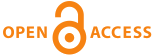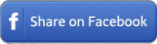Home|Journals|Articles by Year|Audio Abstracts RSS - TOCin any field in Title in Summary in Keywords in Authors in journals

 Original ArticleAJVS. 2016; 51(2): 250-256

##### Deterioration of Beef Meat

Hani E. Youssef, Hossam A. Ibrahim, Sherif A. Ali, Ibrahim A. Samaha.

##### Abstract

A total of 20 freshly slaughtered beef meat samples were collected from different butcher shops at EL- Boheira province. The sample size was about 2 kg. The samples were put in a clean sterile plastic bags and kept in an ice box and transferred to the laboratory with a minimum of delay and kept at chilling condition at 3-4 co until examined at different times of storage , namely after ( zero , 24 ,48 , 72 and 96 hours) of storage. Each sample was divided into 5 pieces, each piece was about 400 gram, each piece was examined for spoilage indices (spoilage markers) and microbiologically. The results revealed that the pH value of the examined beef meat varied from 6.2 to 6. 8, with a mean value of 6.5ï¿½0.3, at Zero Time, 5.6 to 5.9, with a mean value 5.8ï¿½0.3, at 24h , 5.7 to 6.1, with a mean value of 5.9ï¿½02, at 48h , 6.2 to6.4, with a mean value of 6.3ï¿½0.2 at 72h, 6.4 to6.7, with a mean value of 6.5ï¿½0.2 at 96h , respectively. While the TVB-N (mg/ 100gm) value of the examined beef meat varied from 4.8 to 9. 6, with a mean value of 7.3ï¿½3.2, at Zero Time, 6.1 to 10.4, with a mean value 9.2ï¿½2.8, at 24h , 9.4 to 13.3, with a mean value of 11.8ï¿½1.9, at 48h , 13.8 to19.2, with a mean value of 16.4ï¿½3.4 at 72h, 21.2 to 24.6, with a mean value of 22.6ï¿½3.4 at 96h , respectively. Also the aerobic plate count (APC) value of the examined beef meat varied from 2.2x104 to 3.1x105, with a mean value of 1.1x105ï¿½1.2x104, at Zero Time, 3.6x104 to 4.2x105, with a mean value 2.3x105ï¿½3.6x104, at 24h , 1.1x105 to 3.2x106, with a mean value of 9.2x105ï¿½2.2x105, at 48h , 1.6x106 to 2.4x107, with a mean value of 5.2x106ï¿½1.3x106 at 72h, 9.6x106 to 1.1x108, with a mean value of 4.6x107ï¿½9.6x106at 96h , respectively. And the Psychrophilic count value of the examined beef meat varied from 1.8x103 to 1.4x104, with a mean value of 7.1x103ï¿½1.6x103, at Zero Time, 4.6x103 to 2.8x104, with a mean value 1.1x104ï¿½9.1x103, at 24h , 2.1x104 to 1.2x105, with a mean value of 6.8x104ï¿½1.3x104, at 48h , 2.8x105 to 7.6x105, with a mean value of 5.2x105ï¿½1.1x105at 72h, 6.3x105to 2.4x106, with a mean value of 1.1x106ï¿½9.6x105at 96h , respectively. While the Enterobacteriaceae count value of the examined beef meat varied from 1.6x103 to 2.1x104, with a mean value of 7.2x103ï¿½1.4x103, at Zero Time, 1.9x103 to 3.1x104, with a mean value 8.1x103ï¿½1.8x103, at 24h , 2.3x103 to 4.6x104, with a mean value of 1.3x104ï¿½9.6x103, at 48h , 5.2x104 to 4.1x105, with a mean value of 1.2x105ï¿½7.2x104at 72h, 2.3x105 to 1.9x106, with a mean value of 8.3x105ï¿½2.6x105 at 96h , respectively. And the Coliform count value of the examined beef meat varied from 1.4x102 to 2.0x104, with a mean value of 5.1x103ï¿½1.2x103, at Zero Time, 1.7x103 to 2.5x104, with a mean value 6.5x103ï¿½1.5x103, at 24h , 1.9x103 to 2.7x104, with a mean value of 1.2x104ï¿½8.5x103, at 48h , 2.5x104 to 2.2x105, with a mean value of 1.1x105ï¿½6.1x104at 72h, 2.1x104 to 1.6x106, with a mean value of 6.3x105ï¿½1.5x105 at 96h , respectively. Also the Staphylococci count value of the examined beef meat varied from 1.2x102 to 2.1x104, with a mean value of 5.2x103ï¿½1.2x103, at Zero Time, 1.5x103 to 2.4x104, with a mean value 6.4x103ï¿½1.5x103, at 24h , 1.7x103 to 2.6x104, with a mean value of 1.2x104ï¿½8.4x103, at 48h , 2.4x104 to 2.2x105, with a mean value of 0.9x105ï¿½6.2x104at 72h, 2.1x104 to 1.5x106, with a mean value of 5.8x105ï¿½1.6x105 at 96h , respectively. And the mould count value of the examined beef meat varied from 1.8x102 to 2.1x103, with a mean value of 9.2x102ï¿½2.3x102, at Zero Time, 2.1x102to 2.8x103, with a mean value 1.1x103ï¿½5.6x102, at 24h , 3.3x102 to 3.9x103, with a mean value of 1.3x103ï¿½9.2x102, at 48h , 1.5x103 to 4.6x103, with a mean value of 2.4x103ï¿½1.1x103at 72h, 1.8x104 to 5.1x104, with a mean value of 3.2x104ï¿½1.3x104 at 96h , respectively. And finally the yeast count value of the examined beef meat varied

Key words: Beef meat - Microbial evaluation - Yeast - pH

Full-text options

 Share this ArticleOnline Article Submission
• ejmanager.com